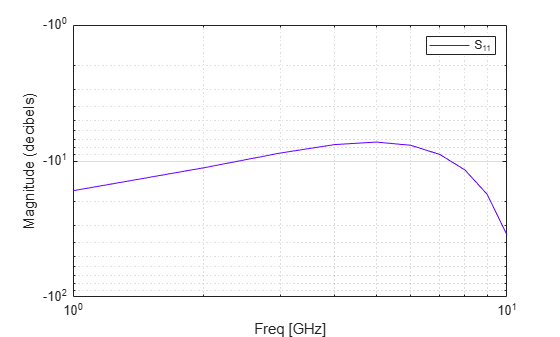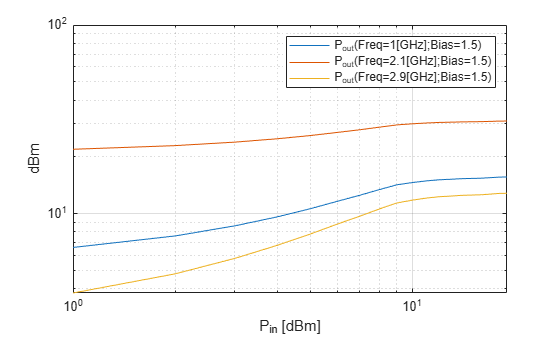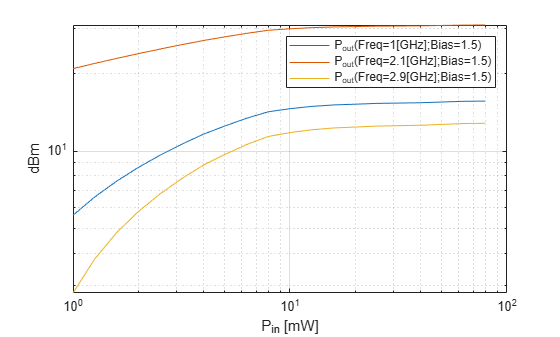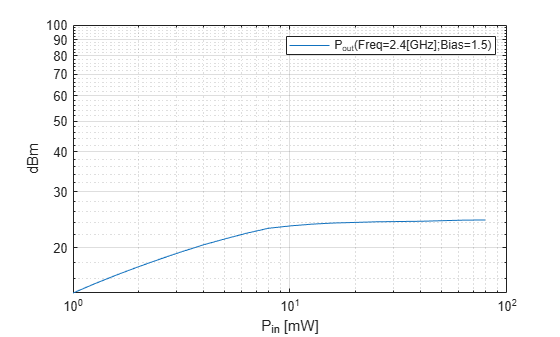loglog

Plot specified circuit object parameters using log-log scale

Description

example

loglog(h,circuitPara) plots the circuit parameter circuitPara from the RFCKT or RF data object h using a log-log scale. You can specify multiple circuit parameters in this syntax.

Note

For all circuit objects except those that contain data from a data file, you must perform a frequency domain analysis with the analyze method before calling loglog.

loglog(h,circuitPara,dataFormat) plots the data of the RFCKT or RF data object using a log-log scale with the specified data format.

example

loglog(___,xAxisPara,xAxisFmt) plots the circuit parameters circuitPara using a log-log scale along with the variables xAxisPara and their corresponding format xAxisFmt. Specify xAxisPara and xAxisFmt arguments after any of the input argument combinations in the previous syntaxes.

loglog(___,opCon,opVal) plots the circuit parameters using a log-log scale with operating conditions opCon and operating values opVal for the circuit object h.

Derive operating conditions for the RFCKT or RF data object h using the getop(h) command

example

loglog(___,Name,Value) plots the data of a RFCKT or RF data object with name-value arguments.

lineseries = loglog(___) returns the line series property object lineseries. This output is the same as the output returned by the MATLAB® loglog function.

Examples

collapse all

Create and analyze a two-wire transmission line RFCKT object.

freq = linspace(1e9,10e9,10);
analyze(h,freq);

Plot S11 using the log-log scale.

lineseries = loglog(h,'S11');

Change the color of the S11 data.

lineseries(1).Color = [0.4 0 1];Create an amplifier object from the specified P2D file type.

Plot the output power of the amplifier.

loglog(h,'Pout')Plot the output power of the amplifier and set the format for Pin in milliwatts.

loglog(h,'Pout','Pin','mW')Plot the output power of the amplifier at 2.4 GHz.

loglog(h,'Pout','Pin','mW','bias',1.5,'Freq',2.4e9)Input Arguments

collapse all

RFCKT or RF data object, specified as a rfckt or rfdata object.

For complete list of RFCKT and RF data objects, see RF Circuit Objects and RF Data Objects.

Format of the data to be plotted, specified as character vector or string scalar. Type listformat(h,circuitPara) command to see the available formats for a specified parameter.

Example: lineseries = semilogy(h,'Pout','dBm')

Valid RFCKT or data object parameter, specified as a character vector or string scalar.

Use listparam(h) for a list of valid parameters for the circuit or data object h.

X-axis variable to plot with the circuit parameters, circuitPara, specified as a character vector or string scalar.

This table shows the commonly used circuitPara and their corresponding xAxisPara values. The function uses the default values listed in the table if you do not specify xAxisPara .

circuitPara ValuexAxisPara Value
Pout, Phase, LS11, LS12, LS21, LS22Pin (default), Freq
S11, S12, S21, S22, NF, IIP3, OIP3, GroupDelay, VSWRIn, VSWROut, GammaIn, GammaOut, FMIN, GammaOPT, RN, TF1, TF2, Gt, Ga, Gp, Gmag, Gmsg, GammaMS, GammaML, K, Delta, Mu, MuPrimeFreq
AM/AM, AM/PMAM

xAxisPara format, specified as a character vector or string scalar. You do not need to specify xAxisFmt when xAxisPara is an operating condition.

This table shows the commonly used xAxisPara and their corresponding xAxisFmt. The function uses the default values listed in the table if you do not specify xAxisFmt .

xAxisPara ValuexAxisFmt Value
PindBm (default), mW, W, dBW
Freq

THz, GHz, MHz, KHz, Hz

By default, xAxisFmt is chosen to provide the best scaling for the given xAxisPara values.

AMMagnitude (decibels) (default), Magnitude (linear)

Example: loglog(h,'Pout','Pin','mW') plots data using a log-log scale for circuit object, h, with xAxisPara set to 'Pin' and xAxisFmt set to 'mW'.

Operating conditions derived from a P2D or S2D file, specified as a string scalar or a character vector.

For some circuit parameters, you can specify a set of frequency or input power values at which the function plots the specified parameter.

For example:

• When plotting large-signal S-parameters as a function of input power, you can specify frequency points of interest using opCon and opVal arguments.

• When plotting large-signal S-parameters as a function of frequency, you can specify input power levels of interest using opCon and opVal arguments.

• When plotting parameters as a function of an operating condition, you can specify both frequency and input power values using opCon and opVal arguments.

Enter the getop(h) command at the command line to get the operating conditions for the RF circuit object h.

Value of the operating conditions specified using the opCon argument, specified as a scalar.

Example: loglog(h,'Pout','Pin','mW','bias',1.5) plots the data using a log-log scale for circuit object, h, with opCon set to 'bias' and value set to 1.5.

Name-Value Arguments

Specify optional comma-separated pairs of Name,Value arguments. Name is the argument name and Value is the corresponding value. Name must appear inside quotes. You can specify several name and value pair arguments in any order as Name1,Value1,...,NameN,ValueN.

Example: loglog(h,'Pout','Pin','mW','bias',1.5,'Freq',2.4)

Frequency value used to plot the data using a log-log scale, specified as the comma-separated pair consisting of 'Freq' and a positive scalar in Hz.

Input power level used to plot the data using a log-log scale, specified as the comma-separated pair consisting of 'Pin' and a scalar in dBm.

Output Arguments

collapse all

Lineseries object, returned as a column vector of object handles.

Tips

• Use the Property Editor ( propertyeditor ) or the MATLAB set function to change Line Properties. The reference pages for MATLAB functions such as figure, axes, and text also list available properties and provide links to more complete property descriptions.

Note

Use the MATLAB loglog function to create a log-log scale plot of parameters that are specified as vector data and are not part of a circuit ( rfckt ) object or data ( rfdata ) object.

• If h has multiple operating conditions, such as from a .p2d or .s2d file, the loglog function operates as follows:

• If you do not specify any operating conditions as arguments to the loglog method, then the method plots the parameter values based on the currently selected operating condition.

• If you specify one or more operating conditions, the loglog method plots the parameter values based on those operating conditions.

• When you use an operating condition for the xparameter input argument, the method plots the parameters for all operating condition values.